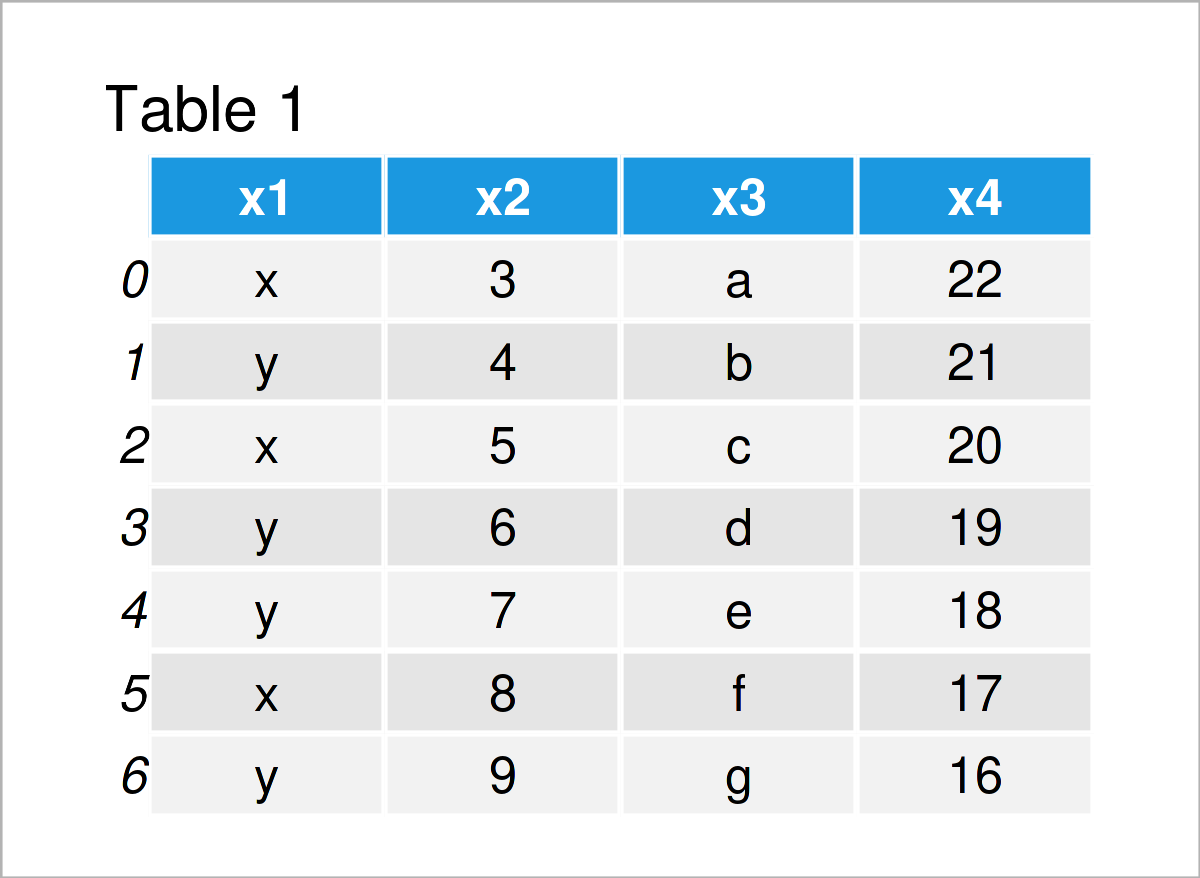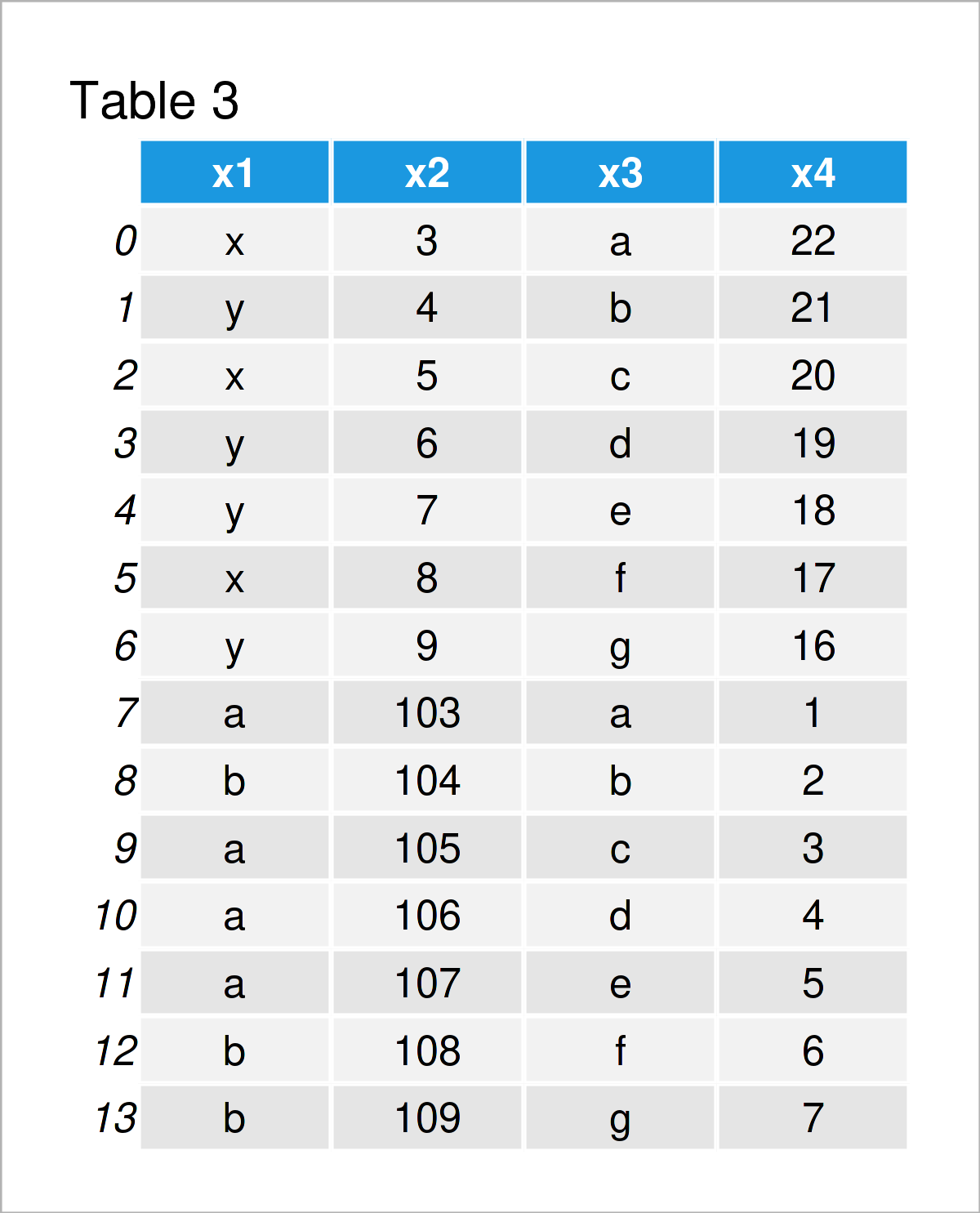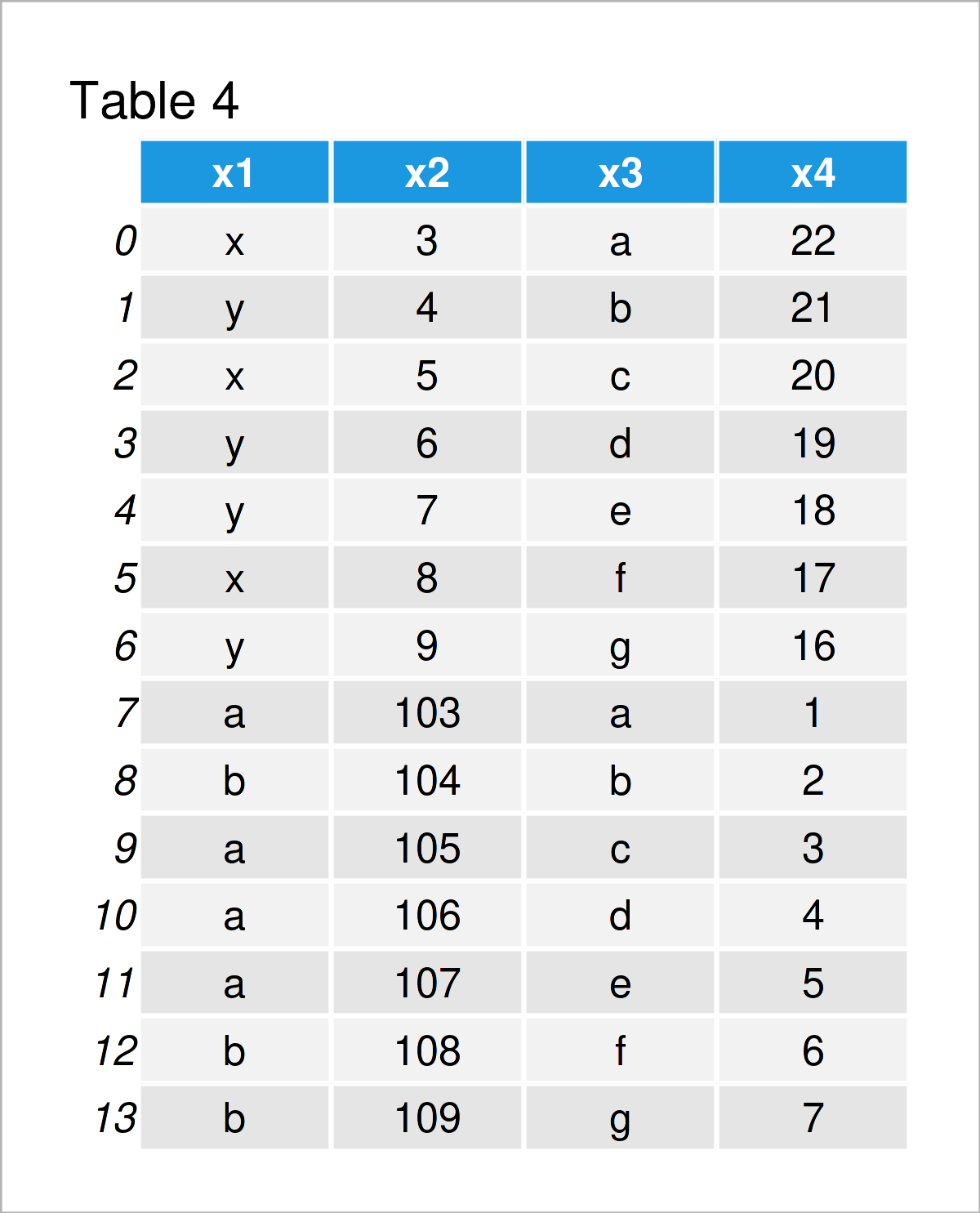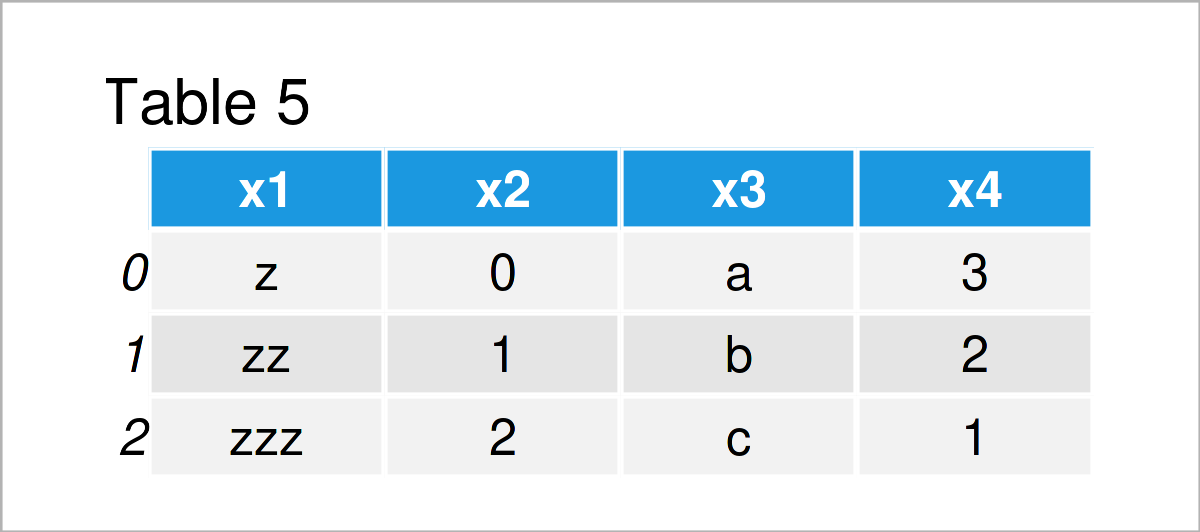# Append pandas DataFrame in Python (4 Examples) | Concatenate & Combine

In this post, I’ll explain how to combine two pandas DataFrames vertically in Python.

## Exemplifying Data & Software Libraries

First, we need to import the pandas library:

`import pandas as pd                                    # Load pandas`

Let’s also create some example data for the examples later on:

```data1 = pd.DataFrame({"x1":["x", "y", "x", "y", "y", "x", "y"],  # Create first DataFrame
"x2":range(3, 10),
"x3":["a", "b", "c", "d", "e", "f", "g"],
"x4":range(22, 15, - 1)})
print(data1)                                           # Print first pandas DataFrame``````data2 = pd.DataFrame({"x1":["a", "b", "a", "a", "a", "b", "b"],  # Create second DataFrame
"x2":range(103, 110),
"x3":["a", "b", "c", "d", "e", "f", "g"],
"x4":range(1, 8)})
print(data2)                                           # Print second pandas DataFrame```The previous tables illustrate the output of the Python console returned after executing the previous Python syntax.

As you can see, we have created two pandas DataFrame objects. Both of these DataFrames contain four columns that are called x1, x2, x3, and x4.

The following examples show how to stack these two DataFrames on top of each other.

## Example 1: Combine Two pandas DataFrames Using append() Function

Example 1 demonstrates how to merge two pandas DataFrames vertically using the append function.

To achieve this, we can use the Python syntax below:

```data_all1 = data1.append(data2,                        # Append DataFrames
ignore_index = True)
print(data_all1)                                       # Print combined DataFrame```As revealed in Table 3, the previous Python programming syntax has constructed a new pandas DataFrame containing the values of both DataFrames.

Note that the values of the first DataFrame are shown at the top, and the values of the second DataFrame have been added at the bottom.

Furthermore, note that the index numbers of our combined DataFrame are ranging from 0 to 13, i.e. the indices of the second DataFrame have been changed.

We were able to achieve this by specifying the ignore_index argument within the append function to be equal to True.

So far, so good! However, there are different alternatives that I want to show you. So keep on reading…

## Example 2: Combine Two pandas DataFrames Using concat() Function

Example 2 shows how to combine two pandas DataFrames using the concat function.

Within the concat function, we have to specify the two DataFrames that we want to union. Furthermore, we can specify that the indices should be reset in the joined DataFrame, and we can avoid sorting:

```data_all2 = pd.concat([data1, data2],                  # Append DataFrames
ignore_index = True,
sort = False)
print(data_all2)                                       # Print combined DataFrame```As you can see in Table 4, the previous Python syntax has created exactly the same pandas DataFrame as Example 1. Whether you prefer to use the append or the concat function is most of the time a matter of taste.

The previous examples have been quite straightforward. Let’s create some more complex data scenarios…

## Example 3: Append Multiple pandas DataFrames

In Example 3, I’ll explain how to append not only two DataFrames, but even three DataFrames on top of each other.

For this, we first have to create a third example DataFrame:

```data3 = pd.DataFrame({"x1":["z", "zz", "zzz"],         # Create third pandas DataFrame
"x2":range(0, 3),
"x3":["a", "b", "c"],
"x4":range(3, 0, - 1)})
print(data3)                                           # Print third pandas DataFrame```In Table 5 you can see that we have managed to construct another pandas DataFrame using the previous Python code.

In the next step, we can apply the concat command once again (as we already did in Example 2). However, this time we are specifying three data sets within the concat function instead of two:

```data_all3 = pd.concat([data1, data2, data3],           # Append DataFrames
ignore_index = True,
sort = False)
print(data_all3)                                       # Print combined DataFrame```In Table 6 it is shown that we have created a pandas DataFrame that contains the values of multiple input DataFrames.

You can use this code to add even more DataFrames. No matter if you want to add four, five, six, or seven pandas DataFrames to your final output. You can simply insert all these DataFrames within the concat function.

## Example 4: Combine pandas DataFrames with Different Column Names

Another problem that often occurs when combining pandas DataFrames in python is that the variables are called differently, or that one of the DataFrame consists of more columns than the other DataFrame.

In Example 4, I’ll demonstrate how to concatenate pandas DataFrames with different column names.

For this, we have to create one more pandas DataFrame:

```data4 = pd.DataFrame({"x1":["a", "b", "c"],            # Create fourth pandas DataFrame
"x3":["d", "e", "f"],
"x5":["g", "h", "i"]})
print(data4)                                           # Print fourth pandas DataFrame```As shown in Table 7, we have created a new pandas DataFrame that contains the variables x1, x3, and x5.

Note that it does not contain the variables x2 and x4 that are contained in our first example DataFrame. Furthermore, it contains the variable x5 that does not exist in our first example DataFrame.

If we want to append two data sets with different column names, we can still apply the concat function as we already did in Examples 2 and 3:

```data_all4 = pd.concat([data1, data4],                  # Append DataFrames
ignore_index = True,
sort = False)
print(data_all4)                                       # Print combined DataFrame```As shown in Table 8, we have created a combined version of our first and our fourth example DataFrames. As you can see, the concat function has inserted NaN values in the columns that did exist only in one of the input DataFrames.

Looks good!

## Video, Further Resources & Summary

Have a look at the following video on my YouTube channel. In the video, I demonstrate the examples of this tutorial.

Please accept YouTube cookies to play this video. By accepting you will be accessing content from YouTube, a service provided by an external third party.If you accept this notice, your choice will be saved and the page will refresh.

In addition, you may want to read the other articles on my website.

In this article you have learned how to append two pandas DataFrames in Python. In case you have additional questions, please let me know in the comments section.

Subscribe to the Statistics Globe Newsletter